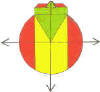DMD   EDU-HOME

WELCOME TO THIS PAGE INTENDED FOR STUDENTS AT THE UNIVERSITY OF THE WESTERN CAPE REGISTERED  FOR THE MODULE QSF 131:

W5 L3

A. CLASS RE-TEST 1:

1.           Calculate:

If 1.42 million South Africans represent 2.75% of its population, then:

1.1           2.5% of the SA population equals: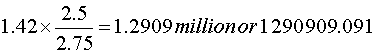1.2       125% of the SA population equals: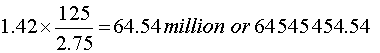(4)

2      2.1   Is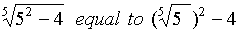? Give a reason(s) for your answer.

2.2   Write each of the expressions in 2.1 in words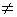-2.09

2.2 Write in your own words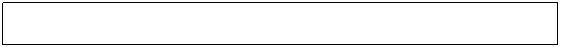(4)

3.  L1 and L2 are two straight line graphs.  What does the intersection point X indicate?4. Determine with your calculator: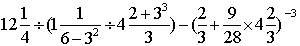(3)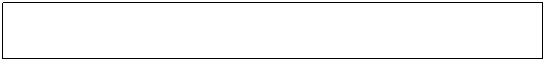10% of the students in a college eat  sweets during the weekend, but of these only 10% eat chocolate. What percentage of the college students eat chocolate ANS 1% (BUT DON’T SOME EAT CHOCS AND NOT SWEETS?)

B TABLE

C: PATTERN :  319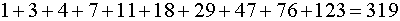7th TERM × 11 = 29 × 11 = 319

(RULE FOR MULTIPLICATION BY 11)

7th TERM =    5th TERM                           +               6th TERM

=  (3rd TERM + 4th TERM) + (4th TERM + 5th TERM)

=  (1st TERM + 2nd TERM) + (2nd  TERM + 3rd  TERM) + ( 2nd TERM                                    + 3rd TERM) + etc…..A group of 24 university students decide to go on a weekend outing of two days. Their expenses will be based on a total of 628 kilometres of traveling, and the following:

·         R580  daily rental for a 20-seater bus plus R1.75 per kilometre;

·         R10.07 per litre of petrol; (Note: Assume 1 litre of petrol covers 9.5 km)

·         R275 per day to hire each of the required 4 bungalows.

·         R330 daily rental plus R1.25 per kilometer for a double cab.

The students all agree  to pay as follows:

·                     The total expense is divided equally amongst themselves;

·                     Two drivers chosen from themselves,  each pay  5.5% less than their mates;

·                     Those who withdraw have to pay R100 penalty fee.

Calculate the total amount each one has to pay, if three of them withdraw and decide not to join the weekend outing. (If necessary, use the back of this page for your calculations.)

MINI-BUS                   PETROL                  BUNGALOWS                4x42×580                     2×628/9.5×R10.07            4×2×R275                  2×330

+                                                                                                       +

1.75×628                                                                                         1.25×628

TOTAL COSTR2 259 + R1331.36 + R2 200 + R1 445

=R7 235.36

THREE DON’T GO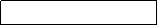R7 235.36 –R300

24 HAVE TO PAY

LET x be the price 19 pay, then 2 will pay 2×x×0.945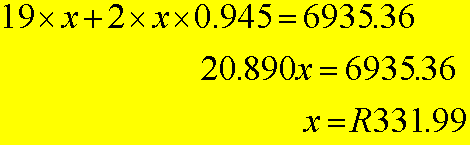1. USAIN BOLT :

PROBLEM 1

In the recent 2008 Olympic games held in the Bird’s Nest stadium in Beijing, the Jamaican sprinter Usain Bolt ran the 100 metres in 9.69 seconds to clinch the gold medal for this event.

(a) Estimate his average speed over this distance in km per hour.

(b) Express his average speed over this distance in km per hour.

Solution

(a) One has a good idea of 20, 60 and 80 km/hour (stemming from our car driving experiences). Hence as good estimate would be 30-40 km/hr.

(b) Time needed to cover 100 metres  = 9.69 seconds

Since 1 km is ten times 100 metres, time taken to complete 1 km = 96.9 seconds = 1.615 minutes

(divide  by 60, since 60 secs = 1 minute)

Therefore, the distance covered in 1 hour = 60 divided by 1.615 = 37.15 km

Therefore Bolt’s average speed = 37.15 km/hour

1. WHICH IS BETTER?

PROBLEM 2

A special type of engine oil treatment is sold for R119 for 350 ml at a particular store. The same store also sells a 500 ml container of the same thing for R149. Which is the better buy?

Partial Solution:

Work out the respective prices per ml for each OR

Work out the respective quantities per rand.

DO YOURSELF

In the World Athletics Championship competition  held …….., Germany on Sunday 16 August 2009, the Jamaican sprinter Usain Bolt ran the 100 metres in ……….. seconds to clinch the gold medal for this event. Determine:

(1)   By how many seconds did Bolt improve his previous world record set a year ago? (0.11 secs)

(2)   His speed in km/h over the distance. (37.5782881 km/h)

(3)   What is the percentage decrease in the time over the distance? (1.148%)

(4)   What is the percentage decrease in his speed over this distance? (1.152%)

1. LETTUCES / HAMMERING / JOURNEY: THANDI

PROBLEM 3

When laying a wooden floor of a room, a workman hammered in nails at an average rate of two nails every 2 minutes and 15 seconds. In total, 550 nails were needed to do the job.  If the average time taken to hammer in the nails becomes 0.125 seconds less each time after the first 10 had been hammered in, calculate the total time in hours taken to hammer in ALL the nails?

Solution:

Time to hammer in 10 nails = 2.25 × 5 = 11.25 minutes

Time to hammer in 11th nail = (½ of 2.25×60 – 0.125) secs = 67.375 secs  = a

d = 0.125 secs

n = 540

Thus           T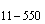=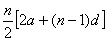=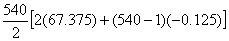secs

=   18191.25 secs

=   5.053125 hours

=   5 hours 3 mins 11.25 secs

©  DESMOND DESAI, DMD EDU-HOME, 2010

All rights reserved. Designed and created by Desmond Desai, South Africa. This page is protected by Copyright. No part of this page may be reproduced, stored in a retrieval system, or transmitted in any form of by any means, electronic, mechanical, photocopying, recording or otherwise, without the prior written permission of the copyright holder.